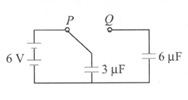Capacitors and capacitance
Question

# In the circuit shown, a capacitor of capacitance $3 \mu F$ is charged form a battery of emf 6 V with switch connected to terminal P. The switch is now connected to Q. This charges the $6 \mu F$ capacitor from the $3 \mu F$ one. What is the new potential difference across combination?Moderate
Solution

## Initially at switch position Q, by conservation of charge, the voltage V across the two capacitors is thus given by$q={q}_{1}+{q}_{2}=C{V}_{1}+{C}_{2}V=\left({C}_{1}+{C}_{2}\right)V$or $18=\left(3+6\right)V$or $V=\left(18/9\right)=2V$

Get Instant Solutions